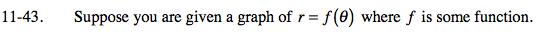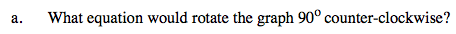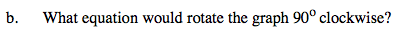### Home > PC > Chapter 11 > Lesson 11.1.3 > Problem11-43

11-43.
1. Suppose you are given a graph of r = f (θ) where f is some function. Homework Help ✎

1. What equation would rotate the graph 90°counter-clockwise?

2. What equation would rotate the graph 90°clockwise?$\textit{r}=\textit{f}(\theta-90\degree)=\textit{f}(\theta-\frac{\pi}{2})$How is this answer going to be the same as and/or different from the answer to part (a)?